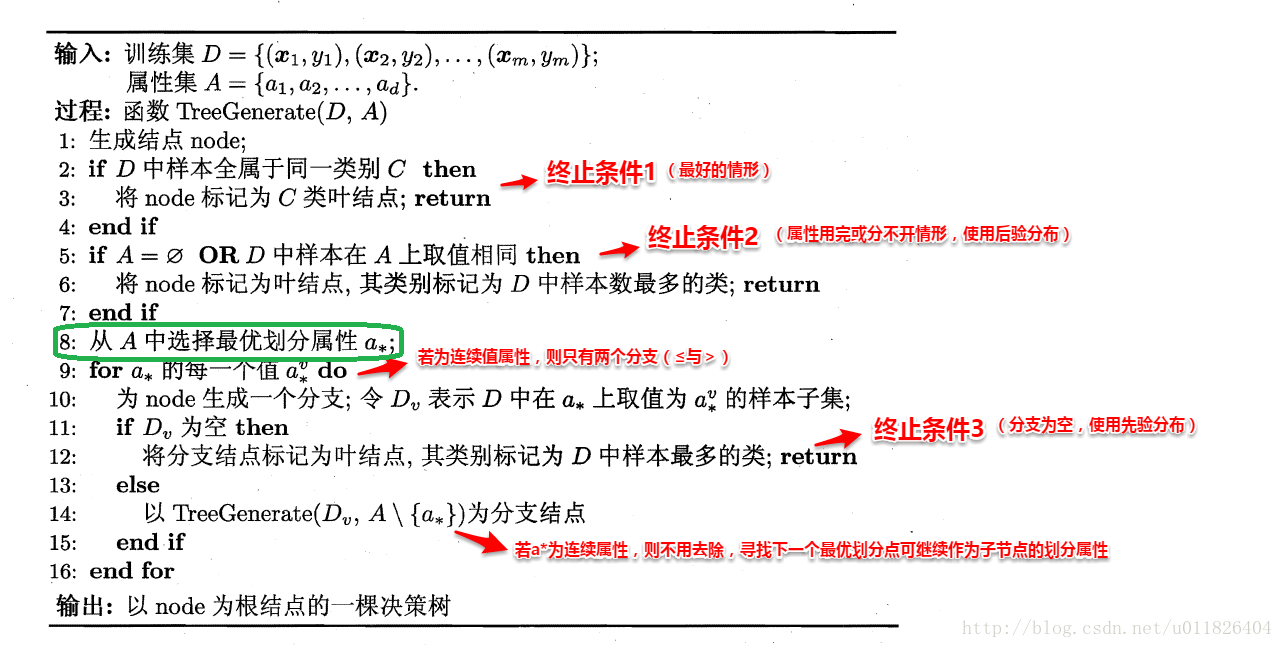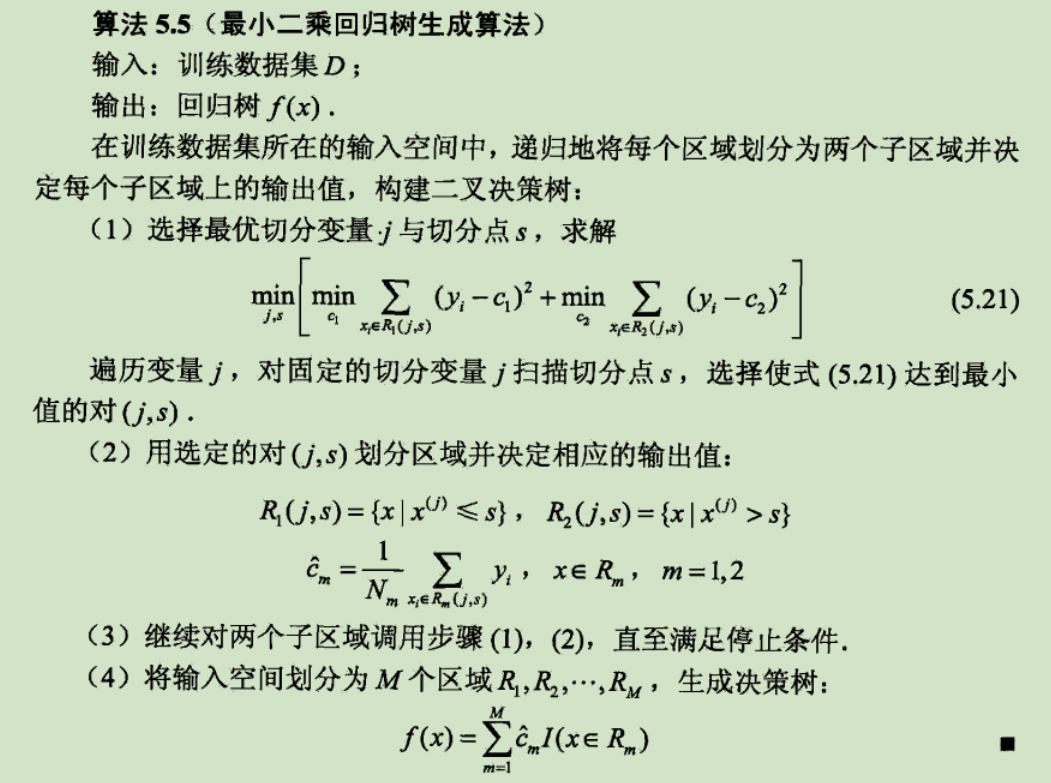# 决策树(分类)

2019-01-10

## 1 理论基础

### 1.1 信息熵

$Ent(D)=-\sum\limits_{k=1}^{|y|}P_k\log_2 P_k$

$Ent(D)$的值越小，则$D$的纯度越高。$Ent(D)$的最小值为0，最大值为$\log_2 |y|$

### 1.2 信息增益

$Ent_a(D)=\sum\limits_{i=1}^{v}\dfrac{|D^i|}{|D|}Ent(D^i)$

$Gain(D,a)=Ent(D)-Ent_a(D)=Ent(D)-\sum\limits_{i=1}^{v}\dfrac{|D^i|}{|D|}Ent(D^i)$

### 1.3 信息增益率

ID3算法存在一个问题，就是偏向于多值属性，C4.5使用信息增益率（gain ratio）的信息增益扩充，来克服这个偏倚。信息增益率的计算公式如下：

$Gain\_ratio(D,a)=\dfrac{Gain(D,a)}{IV(a)} \\ IV(a)=-\sum\limits_{i=1}^{v}\frac{|D^i|}{|D|}\log_2 \frac{|D^i|}{|D|}$

C4.5选择具有最大增益率的特征作为分裂特征。[网上大部分的说法]

### 1.4 Gini指数

$Gini(D)=1-\sum\limits_{k=1}^{|y|}P_k^2$

$Gini(D)$反映了从数据集$D$中随机抽取两个样本，其类别标记不一致的概率。因此，$Gini(D)$越小，数据集$D$的不纯度越小，相反纯度越高。

$Gini\_index(D,a)=\sum\limits_{i=1}^{v}\frac{|D^i|}{|D|}Gini(D^i)$

## 2 决策树算法1. 当前结点包含的样本全属于同一个类别，无需划分；
2. 当前的属性集为空，或是所有样本在所有属性集上取值相同，无法划分。此时，我们将当前结点标记为叶子结点，并将其类别设定为该结点所包含样本最多的类别
3. 当前结点包含的样本集合为空，不能划分。我们同样将当前结点标记为叶结点，但将其类别设定为其父结点所含样本最多的类别

### 2.1 ID3

1. 计算整个数据集的信息熵；
2. 分别计算以某个属性进行划分数据集时的信息熵，并联合整个数据集的信息熵计算信息增益；
3. 选取最大信息增益的属性最为分裂点；

ID3算法的优点是：算法的理论清晰，方法简单，学习能力较强缺点是：只对比较小的数据集有效，且对噪声比较敏感，当训练数据集加大时，决策树可能会随之改变。

### 2.2 C4.5

C4.5是机器学习算法中的另一个分类决策树算法，它是基于ID3算法进行改进后的一种重要算法，相比于ID3算法，改进有如下几个要点：

1. 信息增益率来选择属性；
2. 在决策树构造过程中进行剪枝，因为某些具有很少元素的结点可能会使构造的决策树过拟合（Overfitting），如果不考虑这些结点可能会更好；
3. 对非离散数据也能处理；
4. 能够对不完整数据进行处理。

C4.5的算法流程和ID3类似，只不过是计算信息增益率，然后选择信息增益率大的进行分裂。

C4.5算法有如下优点产生的分类规则易于理解，准确率较高。缺点是：在构造树的过程中，需要对数据集进行多次的顺序扫描和排序，因而导致算法的低效。

### 2.3 CART(分类回归树算法)

CART算法是一种二分递归分割技术，把当前样本划分为两个子样本，使得生成的每个非叶子结点都有两个分支，因此CART算法生成的决策树是结构简洁的二叉树。由于CART算法构成的是一个二叉树，它在每一步的决策时只能是“是”或者“否”，即使一个feature有多个取值，也是把数据分为两部分。

CART算法由以下两步组成：

1. 决策树生成： 就是递归地构建二叉决策树的过程，对回归树平方差最小化准则，对分类树基尼指数最小化准则，进行特征选择，生成二叉树；
2. 决策树剪枝： 用验证数据集对已生成的树进行剪枝并选择最优子树，这时损失函数最小作为剪枝的标准。

CART决策树既可以用于分类也可以用于回归，分类树与回归树的一个区别是：如果目标变量是离散型变量则用分类树，如果目标变量是连续型变量则用回归树

#### 2.3.1 分类树

$Gini(p)=2p(1-p)$

$Gini(D)=1-\sum\limits_{k=1}^{|y|}P_k^2$

$Gini(D)=2p(1-p)$

$D_1=\{(x,y)\in D|A(x)=a\}, D_2=D-D_1$

$Gini(D,A=a)=\dfrac{|D_1|}{D}Gini(D_1)+\dfrac{|D_2|}{D}Gini(D_2)$

CART分类树算法：

1. 设结点的训练数据集为$D$，计算现有特征对该数据集的Gini系数。此时，是 对每一个特征$A$，对其可能取的每个值$a$，根据样本点对$A=a$的测试为“是”或 “否”将$D$分割成$D_1$$D_2$两部分，计算A=a时的Gini系数。
2. 在所有可能的特征$A$以及它们所有可能的切分点$a$中，选择Gini系数最小的特征及其对应的切分点作为最优特征与最优切分点。依最优特征与最优切分点，从现结点生成两个子结点，将训练数据集依特征分配到两个子结点中去。
3. 对两个子结点递归地调用步骤l~2，直至满足停止条件。
4. 生成CART分类树。

#### 2.3.2 回归树## 3 连续值处理

1. 将节点Node上的所有数据样本按照连续型属性$A$的具体取值，由小到大进行排列，得到属性$A$的属性值取值序列$(x_1^A,...,x_N^A)$
2. 在序列$(x_1^A,...,x_N^A)$中共有$N-1$种二分方法，即共产生$N-1$个分隔阈值。对于第$i$种二分方法，其二分阈值$\theta_i=\dfrac{x_i^A+x_{i+1}^A}{2}$。它将该节点上的数据集划分为2个子数据集$(x_1^A,...,x_i^A)$$(x_{i+1}^A,...,x_N^A)$。计算此种二分结果下的信息增益或基尼系数。
3. 分别计算$N-1$种二分结果下的信息增益或基尼系数，选取信息增益最大或基尼系数最小的二分结果作为对属性A的划分结果，并记录此时的二分阈值。

## 4 决策树剪枝

### 4.2 后剪枝

1. 对于完全决策树中的每一个非叶子节点的子树，我们尝试着把它替换成一个叶子节点，该叶子节点的类别我们用子树所覆盖训练样本中存在最多的那个类来代替，这样就产生了一个简化决策树；
2. 然后比较这两个决策树在测试数据集中的表现，如果简化决策树在测试数据集中的错误比较少，那么该子树就可以替换成叶子节点
3. 该算法以bottom-up(自底向上) 的方式遍历所有的子树，直至没有任何子树可以替换使得测试数据集的表现得以改进时，算法就可以终止。

## 5 总结

• 计算简单，易于理解，可解释性强；
• 比较适合处理有缺失属性的样本；
• 能够处理不相关的特征；
• 在相对短的时间内能够对大型数据源做出可行且效果良好的结果。

• 容易发生过拟合（随机森林可以很大程度上减少过拟合）；
• 忽略了数据之间的相关性；
• 对于那些各类别样本数量不一致的数据，在决策树当中，信息增益的结果偏向于那些具有更多数值的特征（只要是使用了信息增益，都有这个缺点，如RF）。